A quick explanation of the Mandelbrot set

The Mandelbrot set is perhaps the most famous fractal. When Benoît Mandelbrot first saw an image of the set that would one day bear his name, he was probably looking at a black and white print-out that took several minutes to complete.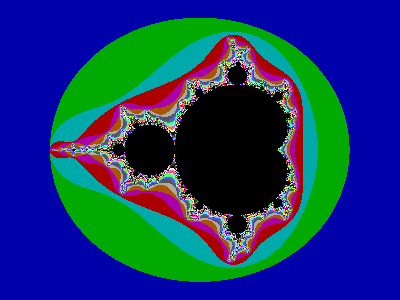The Mandelbrot set, in color. I don’t remember if this was under DOS or Windows 95.

At first he thought he had made a mistake. So he checked the math and found that, making allowances for the available numerical precision, the print-out was correct.

The Mandelbrot set is the set of all so-called “complex” numbers that meet Mandelbrot’s simple arithmetic criterion. To make an image of the Mandelbrot set in a given region of the “complex plane,” the computer assigns a complex number to each pixel of the available display area. Then, for each pixel of the display area, the computer determines whether the assigned number meets or doesn’t meet Mandelbrot’s criterion.

If the number meets the criterion, the corresponding pixel is painted black, usually. If it doesn’t meet the criterion, the corresponding pixel is painted white, or some color other than the one used for the numbers that do meet the criterion.

The color for the pixels corresponding to numbers that don’t meet the criterion is more an artistic matter than a mathematical matter. Mathematicians are for the most part only interested in the numbers that do meet the criterion.

Here’s the criterion: take the number, call it c. Assign z = c. Multiply z by itself and add c, reassign that result to z, and once again multiply z by itself and add c. Keep doing that, or, better yet, have your computer do it.

After enough iterations of that, you will see either that z gets farther and farther away from zero, or you will see that z settles onto a specific single value or a cycle of values that are not that far away from zero. If z settles, then c is in the Mandelbrot set.

For example, take c = 2. That gives us the sequence 2, 6, 38, 1446, 2090918, 4371938082726, 8919542782688501670, etc. These numbers quickly get out of the range a computer can represent. They “escape to infinity,” a mathematician might say. Therefore 2 is not in the Mandelbrot set.

Now compare c = −7/4. That gives us −7/4, 21/16, −7/256, −114639/65536, 5625907553/4294967296, etc. The denominators are getting bigger, but the values circle around zero, staying in the range between −7/4 and 21/16, as the numeric approximations demonstrate: −1.75, 1.3125, −0.02734375, −1.7492523193359375, 1.3098836767021567, etc.

A mathematician might say this is a “stable orbit” or something like that. Definitely not “escape to infinity.” Therefore −7/4 is in the Mandelbrot set.

Note that c doesn’t have to be a “purely real” number like 2 or −7/4. It can be a “purely imaginary” number like the principal square root of −1 or it can be a complex number like −1/2 + (−3)/2.

A complex number has a “real” part and an “imaginary” part. If z is a complex number, it can be expressed as z = x + yi, where x and y are purely real numbers and i is the number such that i² = −1. Note that (−i)² = −1 also.

If z = x + yi, then the function Re(z) = x and the function Im(z) = y (or yi, maybe, I’m not sure). Thus the x and y of a complex number can be readily mapped to the x and y coordinates of the 2-dimensional plane.

Complex number arithmetic is actually not all that complicated, just remember that i² = −1. It should still make us grateful for computers.

And we need hundreds if not thousands of arithmetic calculations in order to draw an image of the Mandelbrot set even at a low resolution like 256 pixels per unit interval.

Looking at an image of the Mandelbrot set at a low resolution and not zoomed in, we might reach the incorrect conclusion that the numbers of the Mandelbrot set are not actually pathwise connected.

That would mean that to draw a path from a certain number in the Mandelbrot set to another number that is also in the Mandelbrot set the path might be required to go through numbers that are not in the Mandelbrot set. For example, getting from point A to point B (both painted black and labeled in red in the following image) seems to require going through several points not in the Mandelbrot set (painted various shades of blue).

But I’m not demanding the path be a straight line. Somehow, mathematicians were able to prove that each number in the Mandelbrot set is indeed pathwise connected to any other number in the Mandelbrot set.

The proof of the pathwise connection is more advanced than I can understand, but enough experts have looked at it over the decades that I feel confident any mistake in the proof would have been found by now.

The path connecting two numbers of the Mandelbrot set might be winding and tortuous, but it exists, and if your program can zoom in close enough, you can see it. In the example below, I sketch a possible path, then I shifted the winding red line so that you can get a better sense of the path.

Clearly the line I’ve drawn doesn’t have enough twists, it’s too straight even though it’s not an actual straight line.

As you can see, zooming in on the image of the Mandelbrot set reveals copies of the overall shape zoomed out.

Mathematicians have also proved that these copies recur infinitely, and they’re all connected to the “main shape” with lines that often wind around. The only limitation for us to see those copies is in our programs and displays.

For some values of c, the number of necessary calculations is too much for any computer. Therefore our computers need to be programmed with an iteration maximum. If z has not escaped to infinity when the iteration maximum is reached, then the computer is programmed to assume that the iteration does not escape to infinity.

A value like 256 might be a sufficient iteration maximum up to maybe 8,192 pixels per unit interval. As the user commands the program to zoom in more, it may be necessary to increase the iteration maximum.

The program might automatically raise the iteration maximum, or it might require a command from the user, because there could be a trade-off: more iterations might mean crisper images that take a lot longer to render.

You can tell that an iteration maximum needs to be raised when zooming in when you see that where you expect small copies of the overall shape you instead see more of an anonymous blob.

There was a program called WinFract that you could run on Windows 95, Windows NT, Windows XP and Windows Vista. WinFract had a setting for iteration maximum that you could change as needed, but the program gave no guidance as to whether a higher iteration maximum was necessary.

WinFract doesn’t run on Windows 8 or 10 (I’ve tried) and probably won’t run on Windows 11 either, except maybe through a DOS emulator. Maybe I should write my own program in Java? But that’s another article.

The one Mandelbrot set is closely related to the Julia sets, of which there are infinitely many (though the empty set is also the Julia set for infinitely many values of c).

The criterion for a Julia set is exactly the same as the criterion for the Mandelbrot set, with the crucial difference that c is chosen in advance and does not change through the calculations, and the x and y coordinates of an individual point under consideration are fed directly to z without passing through c.

Here’s a zoom of the Mandelbrot set around −7/4. This is one of the “copies” of the overall shape I was mentioning earlier.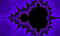The Mandelbrot set zoomed in around −7/4 at 16,384 pixels per unit interval.

Notice that there is a thin straight line that connects this copy to the overall shape. That line is for all values of c such that Im(c) = 0.

And here’s the Julia set for −7/4: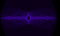The Julia set for −7/4 at 256 pixels per unit interval.

It seems to consist of disconnected broccoli shapes at various sizes. But if we zoom in to 16,384 pixels per unit interval, we might see at least a hint of a straight line connecting the broccoli shapes.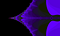The Julia set for −7/4 zoomed in to 16,384 pixels per unit interval.

A much more interesting Julia set is the one for −0.1278 + 0.748i.

This corresponds to the point I’ve labeled z on the Mandelbrot set:

You don’t have to be extremely precise, but do try to aim for the center of the letter.

The images of some Julia sets show multiple Douady rabbits that are connected by lines, not necessarily straight.

This corresponds to: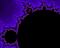A zoom of the Mandelbrot set with an indication of where to find the corresponding Douady rabbits.

Here’s another such Julia set: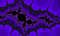The Julia set for −1.77536 + 0.00415i zoomed in to 4,096 pixels per unit interval.

This corresponds to: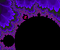A zoom of the Mandelbrot set with an indication of where to find the corresponding Douady rabbits.

What does the Julia set for c look like if c is not in the Mandelbrot set? Depending on how far away c is from the Mandelbrot set, it might look a little bit like a Julia set for a c that is in the Mandelbrot set, but it will not be pathwise connected.

Zooming in, we see that the clusters of numbers that are in this particular Julia set are disconnected from other clusters.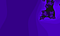Julia set for −0.3 + 0.76i at 2,048 pixels per unit interval.

At 256 pixels per unit interval, we can see the outline of the Douady rabbit, but there is no Douady rabbit to be found. The corresponding point on the Mandelbrot set is not that far away from the coordinates for the Douady rabbit.

I thought the Julia set for c = 2 would be a waste of bandwidth, but it does have a tiny bit of interest to it.

That’s the connection between the Mandelbrot set and the Julia sets. If c is in the Mandelbrot set, then the Julia set for c will be pathwise connected, even if it doesn’t look like it. And if c is not in the Mandelbrot set, then the Julia set for c will not be pathwise connected.

Well, I’ve only scratched the surface of all that is interesting with the Mandelbrot set, and I have not been at all rigorous with the mathematics of it. Hopefully though this is enough to whet your interest in these fractals.

There are programs out there with which you can explore the Mandelbrot set. The programs have different system requirements, and some are better than others. And maybe you can write your own program if none of the available programs suit your system.

is a composer and photographer from Detroit, Michigan. He has been working on a Java program to display certain mathematical diagrams.

More from Alonso Del Arte

is a composer and photographer from Detroit, Michigan. He has been working on a Java program to display certain mathematical diagrams.